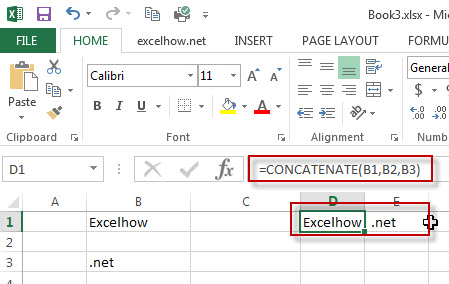## Concatenate columns with Line Breaks (Alt + Enter)

This post will guide you how to combine multiple cells in one or more columns into one cell with line breaks or Alt +enter in Excel. How do I concatenate different values together into one cell with line breaks.

For example, if you are trying to combine three cells (B1,B2,B3) into one(C1) with line breaks or Alt + Enter separating each value. You can refer to the below tutorial to achieve the result.

## Concatenate columns with Line Breaks (Alt + Enter)

To concatenate the cells with line breaks, you should use the concatenate operator in combination with the CHAR(10) or CHAR(13) function. It means that if you want to add line breaks, you need to use the CHAR(10) to insert actual line breaks on Windows or use the CHAR(13) to insert line breaks on MAC system.

To join the cells with line breaks, just do the following steps:

#1 type the following formula into the Cell C1, then press Enter key.

=B1& CHAR(10) & B2 &CHAR(10)&B3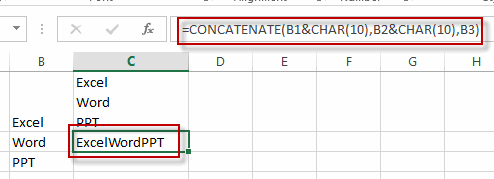or you can also use the CONCATENATE function as below:

=CONCATENATE(B1&CHAR(10),B2&CHAR(13),B3)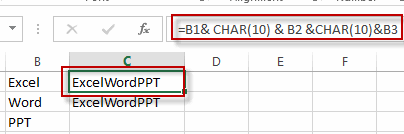2# Select the Cell C1, then go to Home Tab, click Warp Text command under Alignment group.

3# you will see that three cells in column have been concatenated with line breaks or Alt +Enter.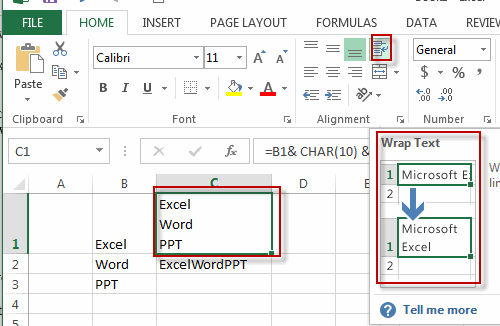### Related Functions

• Excel Concat function
The excel CONCAT function combines 2 or more strings or ranges together.This is a new function in Excel 2016 and it replaces the CONCATENATE function.The syntax of the CONCAT function is as below:=CONCAT (text1,[text2],…)…
• Excel CHAR function
The Excel CHAR function returns the character specified by a number (ASCII Value).The CHAR function is a build-in function in Microsoft Excel and it is categorized as a Text Function. The syntax of the CHAR function is as below:=CHAR(number)….

## How to Add Prefix or suffix to Cell

This post will guide you how to add common prefix or suffix to all cells or the range of cells in your worksheet in Excel. How do I add the same prefix or suffix to a range of cells without any changing the existing numbers or text in the range. How to add prefix or suffix to all cells with Excel VBA Macro.

Assuming that you have a list of data that you want to add common text to the starting position of all cells, of course, you can do it by manually, but it will consume lots of time and it is not a good idea. And you can use the concatenate operator, the CONCATENATE function to create a new Excel formula to add prefix or suffix to the range of cells that you selected. Or you can write a new Excel VBA Macro to achieve the same result.

## Method 1: Using Concatenation Operator to add prefix or suffix

If you want to add prefix or same text to the starting of all cells in your range, you can use the Concatenation operator to build a formula, for example, you want to add same text “excelhow” to all cells in range A1:A4, then you can use the following formula:

`="excelhow"&A1`

Ether this formula in a blank cell, such as: B1, then drag the AutoFill Handle down to other cells to apply this formula.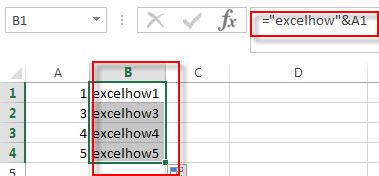If you want to add suffix to all cells with concatenation operator, you can use the following formula:

`=A1&"excelhow"`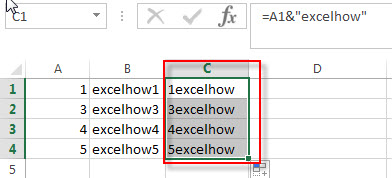You will see that all cells are added the same prefix text in your worksheet.

## Method 2: Using concatenation function to add prefix

To add prefix or suffix text or number to the range of cells with concatenation function, you can wirte down the following formula:

```=CONCATENATE("excelhow",A1)
or
=CONCATENATE(A1,"excelhow")```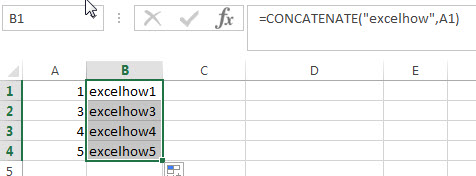## Method 3: Using Excel VBA Macro to add prefix

You can also use the following VBA Macro code to add prefix or suffix in your worksheet in Excel.  Let’s see the below steps:

1# click on “Visual Basic” command under DEVELOPER Tab.2# then the “Visual Basic Editor” window will appear.

3# click “Insert” ->”Module” to create a new module4# paste the below VBA code into the code window. Then clicking “Save” button.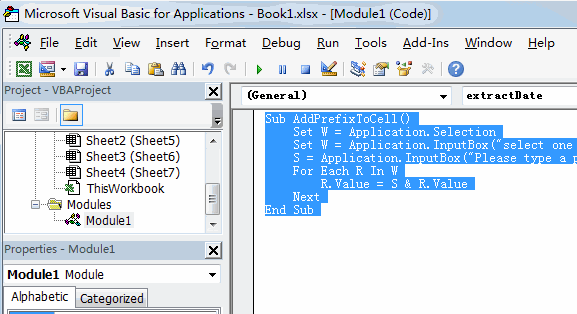```Sub AddPrefixToCell()
Set W = Application.Selection
Set W = Application.InputBox("select one Range that you want to add prefix to each cell:", "AddPrefixToCell", W.Address, Type:=8)
For Each R In W
R.Value = S & R.Value
Next
End Sub```

5# back to the current worksheet, then run the above excel macro. Click Run button.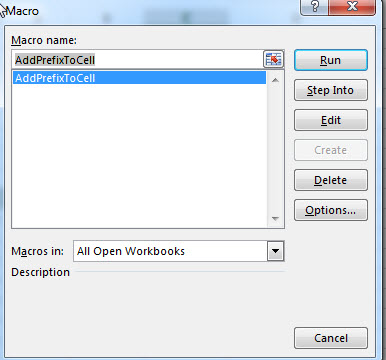6# select a range that you want to add prefix, click OK button, then add one prefix string or character. Click OK button.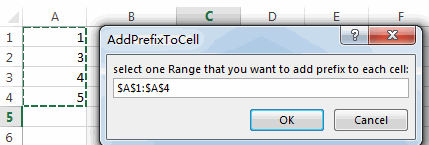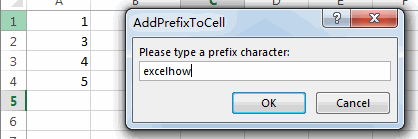7# you will see that the prefix is added to all range of cells.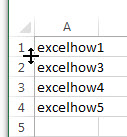If you want to add suffix to a range of cells with VBA macro in Excel, you can use the following VBA macro.

```Sub AddSuffixToCell()
Set W = Application.Selection
Set W = Application.InputBox("select one Range that you want to add suffix to each cell:", "AddSuffixToCell", W.Address, Type:=8)
For Each R In W
R.Value = R.Value & S
Next
End Sub```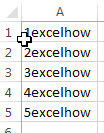### Related Functions

• Excel Concat function
The excel CONCAT function combines 2 or more strings or ranges together.This is a new function in Excel 2016 and it replaces the CONCATENATE function.The syntax of the CONCAT function is as below:=CONCAT (text1,[text2],…)…

## Highlight Duplicate Rows

This tutorial will teach you how to highlight duplicates rows using conditional formatting feature in Excel. In the previous post, we talked that how to change the color of rows based on a certain number or text or begin a specific character in a specified column. And this post will talk that how to highlight entire rows that are duplicates in excel 2016, 2013 or lower version. Or how to change the background color of duplicate rows.

## Highlight duplicate rows in only one column

If you data just have only one column in each rows, then you can following the below steps to highlight duplicate rows:

1# select the range of cells in that column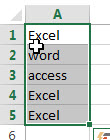2# on the HOME Tab, click Conditional Formatting command under Styles group, click Highlight Cells rules, and then select Duplicate Values.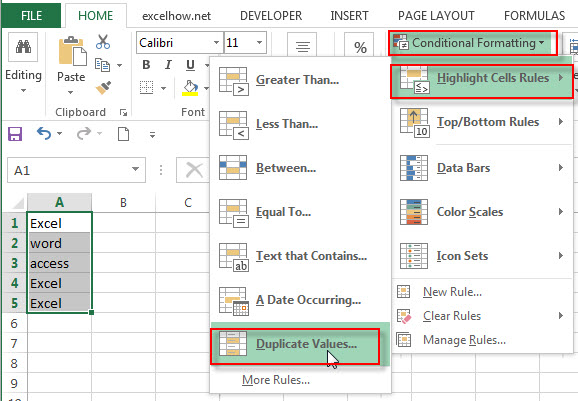3# select Duplicate and Light Red Fill with Dark Red Text from the Format cells that contains box in the Duplicate Values window. Click OK button.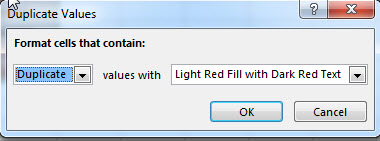4# you will see that all rows which are duplicates are highlighted.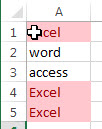## Highlight duplicate rows in multiple columns

Assuming that you want to highlight duplicate rows in a range of cells A2:C4 with conditional formatting, and you need to write a new formula to apply the conditions to find the duplicate rows.

Method 1:

You can create your own formula based on the COUNTIFS function to count duplicated values in each column of your range. So you can use the following formula with COUNTIFS function:

`=COUNTIFS(\$A\$2:\$A\$6,\$A2,\$B\$2:\$B\$6,\$B2,\$C\$2:\$C\$6,\$C2)>1`

Let’s see the below steps:

1# select the range of cells in your table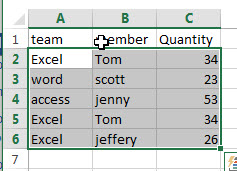2# on the HOME tab, click the Conditional Formatting command under Styles group. Then select New Rules… from the drop-down menu list.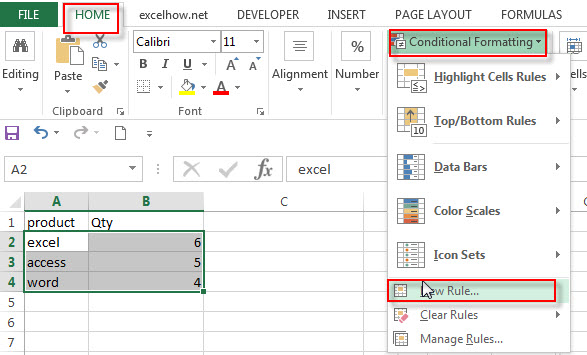3# the New Formatting Rule window will appear.

4# select the Use a formula to determine which cells to format option under Select a Rule Type: box, and then enter the above formula in the Format values where this formula is true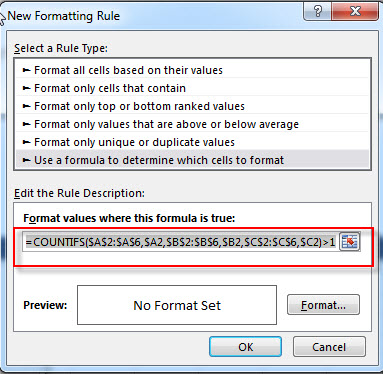5# click the Format… button, then the Format Cells window will appear.

6# in the “Format Cells” window, switch to the Fill tab, choose the background color, and then click OK button. you can also switch to other tabs to tweak the settings as you want.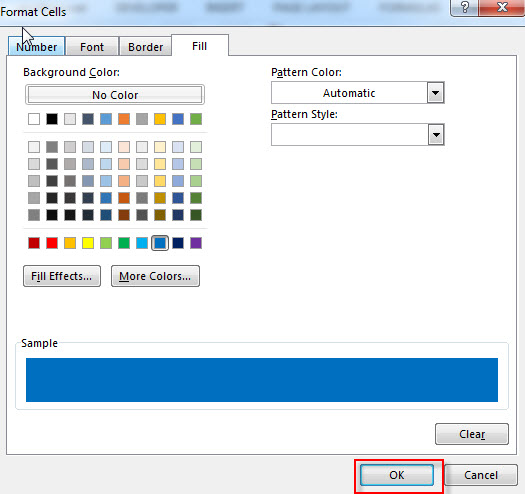7# back in the New Formatting Rule window, you can see a preview of your rows background color. Then click OK button.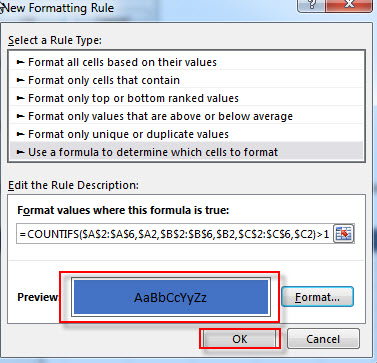8# let’s see the last result.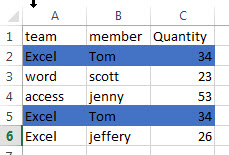If you just want to highlight duplicate rows except for the first occurrences, and you can use the following COUNTIFS formula as the conditional formatting rule.

`=COUNTIFS(\$A\$2:\$A2,\$A2,\$B\$2:\$B2,\$B2,\$C\$2:\$C2,\$C2)>1`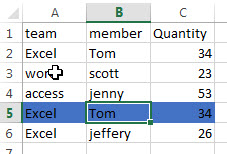Method 2:

You can use the CONCATENATE function or concatenate operator to join all values into one cell in each row, then you just need to check only one cell value in one column to find the duplicate values in the same column. At this time, you can use the COUNTIF function to create a formula, then apply it as the conditional formatting rule to find the duplicate rows. You can do the following steps:

1# create another column where you want to combine all values and call it Joined, such as column D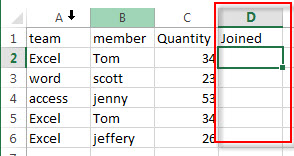2# enter the following formula into the cell D2 to combine columns in each row

`=CONCATENATE(A2,B2,C2)`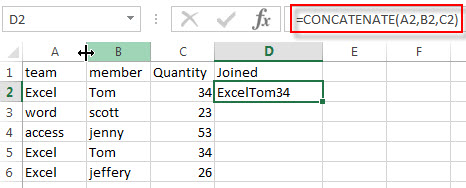3# drag AutoFill Handle down to the rest of cells in column D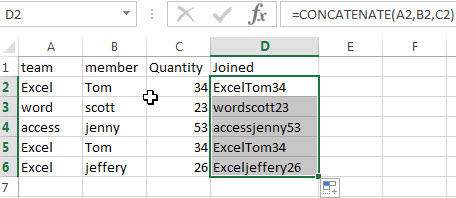4# select the range that you want to highlight duplicate rows including the column D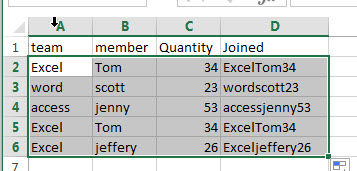5# on the HOME tab-> Styles -> Conditional Formatting, and then click New Rule….

6# select the Use a formula to determine which cells to format option under Select a Rule Type: box, and then enter the following formula in the Format values where this formula is true box.

`=COUNTIF(\$D\$2:\$D\$6,\$D2)>1`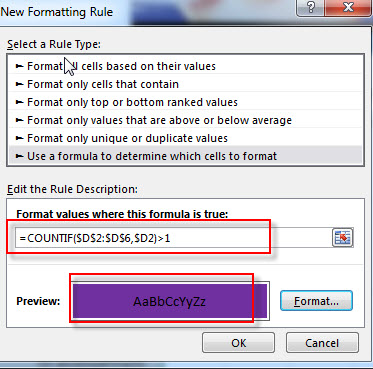7# click the Format… button, then switch to Fill tab, and choose one background color. Click OK.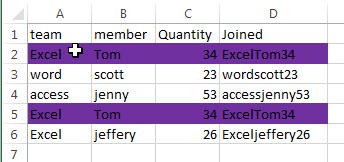8# you can  now delete the column D.

### Related Functions

• Excel Concat function
The excel CONCAT function combines 2 or more strings or ranges together.This is a new function in Excel 2016 and it replaces the CONCATENATE function.The syntax of the CONCAT function is as below:=CONCAT (text1,[text2],…)…
• Excel COUNTIFS function
The Excel COUNTIFS function returns the count of cells in a range that meet one or more criteria. The syntax of the COUNTIFS function is as below:= COUNTIFS(criteria_range1, criteria1, [criteria_range2, criteria2]…)…
• Excel COUNTIF function
The Excel COUNTIF function will count the number of cells in a range that meet a given criteria. This function can be used to count the different kinds of cells with number, date, text values, blank, non-blanks, or containing specific characters.etc.= COUNTIF (range, criteria)…

### Related Posts

• Highlight Rows
You will learn that how to change the color of the entire rows if the value of cells in a specified column meets your conditions, such as, if the value of cells is equal to or greater than a certain number or text values, then excel should be highlight entire rows or change a row color as you need.…
• Find Duplicate Rows
If you want to check the entire row that duplicated or not, if True, then returns “duplicates” value, otherwise, returns “no duplicates”. You can create a formula based on the IF function and the SUMPRODUCT function..…
• Highlight duplicate values
this post will teach you how to highlight duplicate values in the range of cells in excel. Normally, you may be need to identify duplicate values with a range of cells in Excel. And there is one of the fasted way that is using conditional formatting feature in Microsoft Excel……
• Combine Duplicate Rows and Sum the Values
This post will teach you how to combine duplicate rows and sum the corresponding values or calculate numbers in specific column in excel. And how to merge duplicate rows and then sum the values with VBA macro in Excel..…

## How to Combine 3 Cells to Generate One Date

This post explains that how to create a date from 3 different cells in excel. How do I combine 3 general cells to one date cell using excel formula.

Assuming that you have a list of data that contain three columns of data naming: year, month and day. You want to generate a date from combining those three columns into date cell as a standard date.

## Combine 3 Cells to Generate Date

If you want to combine 3 different cells to generate a standard data, you can create an excel formula based on the DATE function. Let’s see the below generic formula:

`=DATE(year, month, day)`

For example,

Suppose that your year value is in Cell A2, the month value is in B2, and the day value is in Cell C2. And then you can write down the following formula using DATE function:

`=DATE(A2,B2,C2)`

You can enter this formula into Cell D2, then press Enter.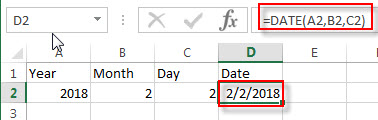You can also use the concatenate operator to combine three cells to one date cell, so you can use “&” to create the below formula:

`=A1&”-“&B1&”-“&C1`

Entering this formula into cell D2, then press Enter.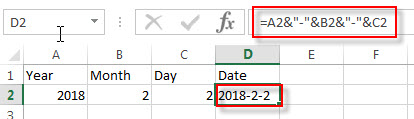### Related Formulas

• Get day name from date
f you want to get the day name from a date in excel, you can use the TEXT function with a specified format code like “ddd” or “dddd”. We can try to use the below TEXT formula in excel…
• Convert date to month and year only in excel
If you want to convert the date to month and year only, you can use “yyyymm” format code within the TEXT function in excel, so you can write down the below TEXT formula:=TEXT(date,”yyyymm”)…
• Convert date to month and day only in excel
If you want to convert the date (mm/dd/yyyy) to month and day only, you can use “mm dd” format code within the TEXT function in excel, so you can write down the below TEXT formula: =TEXT(date,”mm dd”)…

### Related Functions

• Excel DATE function
The Excel DATE function returns the serial number for a date.The syntax of the DATE function is as below:= DATE (year, month, day)…

## How to insert leading zeros to number or text

This post explains that how to insert or add leading zeros in front of a number or text string in excel. How do add the leading zero to the front of the number using Text function in excel.

If you have a list of zip codes or employee ID that were once text with leading zeros, However when you type a zip code like “021103“ in a cell, then you will find excel will truncate it to “21103” immediately. So how to keep leading zeros in the front of zip code in cell.

Assuming that you want to insert leading zeros  to number in Cell A2, then you can try to use the TEXT function to create an excel formula as follows:

`=TEXT(A2,"000000")`

The number format “000000” in TEXT formula will convert the number to a 6 digit number. If a number is greater than zero, then the number will be displayed, otherwise a zero will be displayed. The last result will be stored as Text format.

1# enter the above formula in the Cell C2, then press enter key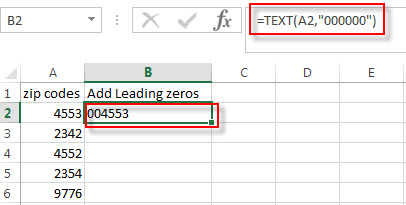2# click AutoFill handle down to all other cells. You will see that all the zip codes in column A are copied to the column C with leading zeros.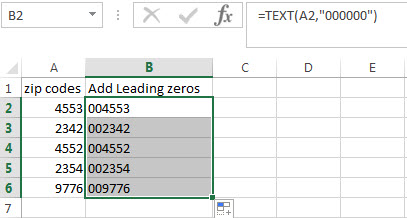You can also use the CONCATENATE function to add the specific digit of leading zeros into each number, such as, adding three leading zeros in the front of the zip code, just use the following formula:

`=CONCATENATE("000",A2)`### Related Functions

• Excel Concat function
The excel CONCAT function combines 2 or more strings or ranges together.This is a new function in Excel 2016 and it replaces the CONCATENATE function.The syntax of the CONCAT function is as below:=CONCAT (text1,[text2],…)…
• Excel Text function
The Excel TEXT function converts a numeric value into text string with a specified format. The TEXT function is a build-in function in Microsoft Excel and it is categorized as a Text Function. The syntax of the TEXT function is as below: = TEXT (value, Format code)…

### Related Posts

• How to keep or remove leading zeros
If you want to keep the leading zeros as you type in a cell, you need to change the cell format to Text before you type the zip code number.You can remove or delete leading zeros in front of the cells with excel VBA macro…
• Count Numbers with Leading Zeros
Suppost you have a list of product ID in your worksheet, and the product ID contains the different number of leading zeros in the front of string. how to preserve the leading zeros while counting numbers. And you can use another Excel function named as SUMPRODUCT.…

## How to add text to the end all cells

In the previous post, we talked that how to add the same text into the beginning of the text in one cell or all selected cells. And this post will guide you that how to add the specified text or characters to the end of all cells in excel. How to create an excel formula to add same text string or characters to the end of text string in one Cell. How to create an excel macro to add specific text to the end of the text in all of cells.

## Add text to the end of all cells with Formula

To add the specified text string or characters to the end of all selected cells in excel, you can use the concatenate operator or the CONCATENATE function to create an excel formula.

For example, if you want to add text “excel” into the end of the text in Cell B1, you can use the following excel formula:

`= B1&" "& "excel"`

OR

`=CONCATENATE(“B1”,””,”excel”)`

You can enter the above formulas in Cell C1, and then drag the fill handle down to other cells in column C and you will see that the specified text “excel” has been added into the end of the text string in B1.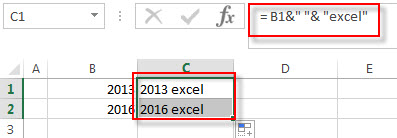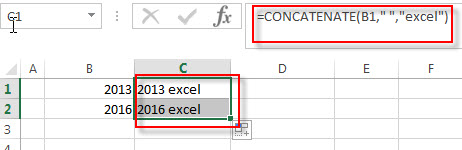## Add text to the beginning of all cells with Excel VBA

You can create a new excel macro to add text string “excel” to the end of text in Cell B1 in Excel VBA, just refer to the below steps:

1# click on “Visual Basic” command under DEVELOPER Tab.2# then the “Visual Basic Editor” window will appear.

3# click “Insert” ->”Module” to create a new module4# paste the below VBA code into the code window. Then clicking “Save” button.

```Sub addTextAtEndCell()
Dim e as range
For each e in Selection
If e.value <> "" Then e.value = e.value & "excel"
Next
End Sub```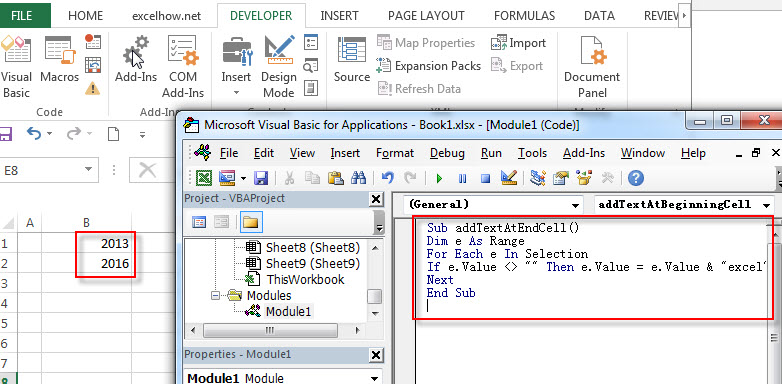5# back to the current worksheet, then run the above excel macro, you will see that the specific text “excel” has been added into the end of the text in all selected Cells.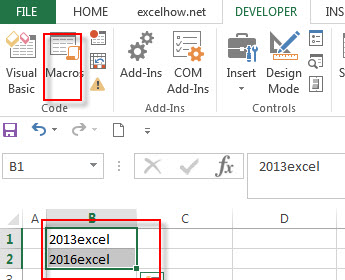### Related Functions

• Excel Concat function
The excel CONCAT function combines 2 or more strings or ranges together.This is a new function in Excel 2016 and it replaces the CONCATENATE function.The syntax of the CONCAT function is as below:=CONCAT (text1,[text2],…)…

## How to add text to the beginning of all cells

This post explains that how to add the specified text or characters to the beginning of all cells in excel. How to create an excel formula to add same text string or characters to the beginning of text string in one Cell. How to create an excel macro to add specific text to the beginning of the text in all of cells.

## Add text to the beginning of all cells with Formula

If you want to add the specific text or characters into the beginning of the text in one cell or all cells, you can create an excel formula based on the concatenate operator or CONCATENATE function.

Assuming that you want to add text “excel” into the beginning of the text in Cell B1, you can write down the following formula:

`="excel"&" "& B2`

OR

`=CONCATENATE(“excel”,””,B1)`

You can enter the above formulas in Cell C1, and then drag the fill handle down to other cells in column C and you will see that the specific text will add the beginning of the text in Cell B1.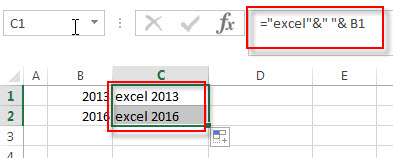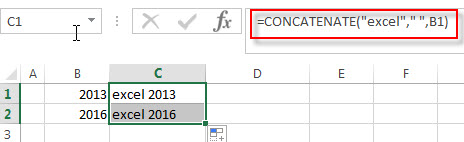## Add text to the beginning of all cells with Excel VBA

You can create a new excel macro to add text string “excel” to the beginning of text in Cell B1 in Excel VBA, just refer to the below steps:

1# click on “Visual Basic” command under DEVELOPER Tab.2# then the “Visual Basic Editor” window will appear.

3# click “Insert” ->”Module” to create a new module4# paste the below VBA code into the code window. Then clicking “Save” button.

```Sub addTextAtBeginningCell()
Dim r As Range
For Each r In Selection
If r.Value <> "" Then r.Value = "excel " & r.Value
Next
End Sub```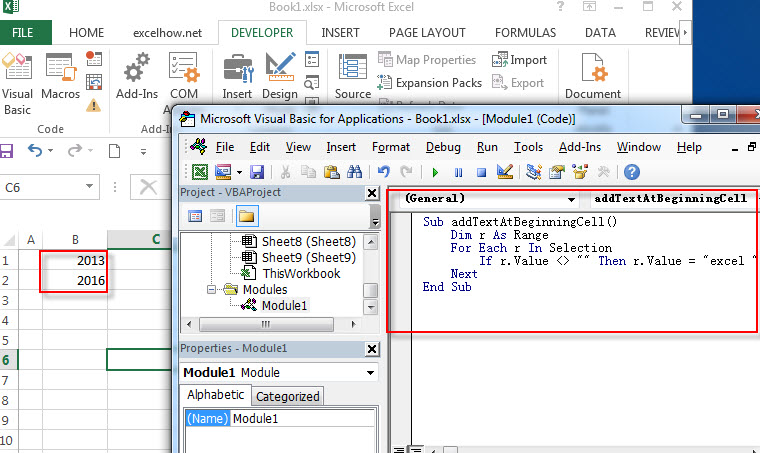5# back to the current worksheet, then run the above excel macro, you will see that the specific text “excel” has been added into the beginning of the text in all selected Cells.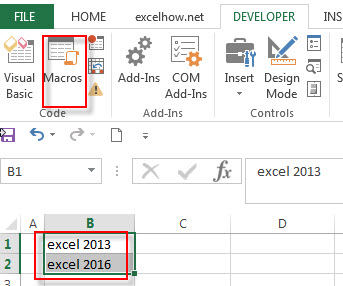### Related Functions

• Excel Concat function
The excel CONCAT function combines 2 or more strings or ranges together.This is a new function in Excel 2016 and it replaces the CONCATENATE function.The syntax of the CONCAT function is as below:=CONCAT (text1,[text2],…)…

## How to combine columns without losing data

This post will guide you how to merge and combine multiple columns without losing any data in excel. How do I merge columns without losing data using Excel Formula. For example, you want to join two columns that contain first name and last name into one column without losing data.

If you want to merge multiple columns that you selected and you can use the “Merge & Center” command, but there is one problem is that it just keep the upper-left most data only. so if you just want to merge multiple columns and don’t care about if the data is deleted or not.

Firstly, Click “Merge & Center” command under Alignment group in Home Tab.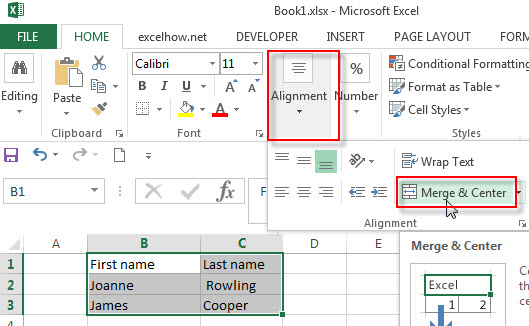then you will get a warning message that is “Merging cells only keeps the upper-left cell value, and discards the other values.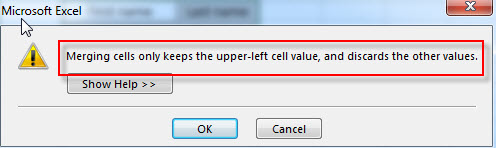## Merge columns without losing data

How to keep all data after merging columns. You can use the concatenate operator or CONCATENATE function to create an excel formula. Assuming that you want to merge column B and C into column D, you can use the following formulas:

`=B2&C2`

OR

`=CONCATENATE(B2, “ “ ,C2)`

You can write the above formula in Cell D2, you will see that both column B and column C have been merged into column D without losing any data.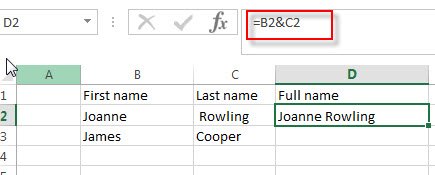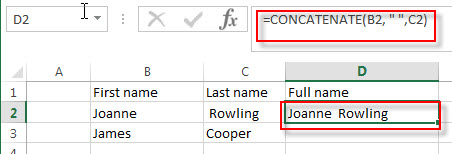Then you can drag the fill handle to other cells in Column D to apply the above formula to merge other cells.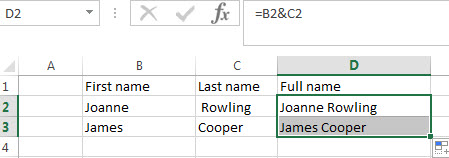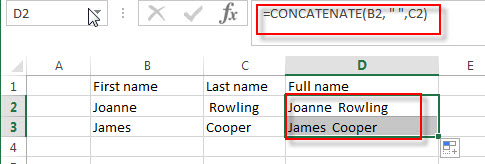### Related Functions

• Excel Concat function
The excel CONCAT function combines 2 or more strings or ranges together.This is a new function in Excel 2016 and it replaces the CONCATENATE function.The syntax of the CONCAT function is as below:=CONCAT (text1,[text2],…)…

### Related Posts

• Combine multiple workbooks into one workbook
If you want to combine multiple workbooks into one workbook, you need to open all workbooks, then determine the workbooks to merge and the destination of workbook.…
• Merge multiple worksheets into one worksheet
How to merge two or more excel worksheet into one worksheet by using some VBA code. You can create a new excel macro to combine multiple worksheets into one worksheet in Excel VBA…

## How to Combine Text from Two or More Cells into One Cell

This post explains that how to combine text from two or more cells into one cell in excel. How to concatenate the text from different cells into one cell with excel formula in excel. How to join text from two or more cells into one cell using ampersand symbol. How to combine the text using the TEXTJOIN function in excel 2016.

## Combine text using Ampersand symbol

If you want to combine text from multiple cells into one cell and you can use the Ampersand (&) symbol. For example, if you want to combine texts in the Range B1:B3 into Cell C1, Just refer to the following steps:

1# Click Cell C1 that you want to put the combined text.

2# type the below formula in the formula box of C1.

`=B1&B2&B3`

3# press Enter, you will see that the text content in B1:B3 will be joined into C1.Note:

If you want to add a space between the combined text, then you can use &” ” & instead of & in the above formula.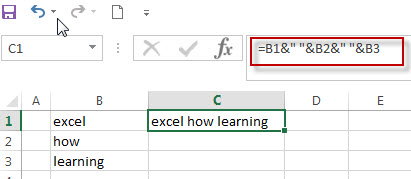And if you want to add a comma to the combined text, just type &”,”& between the combined cells in the above formula.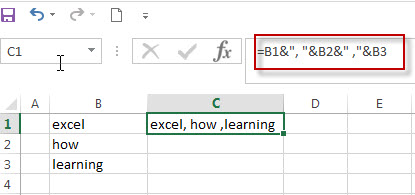If you want to add Line break between the combined cells using Ampersand operator, you should combine with the CHAR function to get the line break. Using the following formula:

`=B1 & CHAR(10) & B2 & CHAR(10) & B3`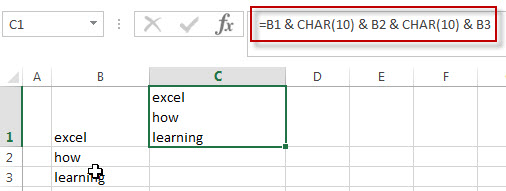## Combine text using CONCATENATE function

If you want to join the text from multiple cells into one cell, you also can use the CONCATENATE function instead of Ampersand operator. Just following the below steps to join combine the text from B1:B3 into one Cell C1.

1# Select the cell in which you want to put the combined text. (Select Cell C1)

2# type the following formula in Cell C1.

`=CONCATENATE(B1,B2,B3)`

3# press Enter to complete the formula.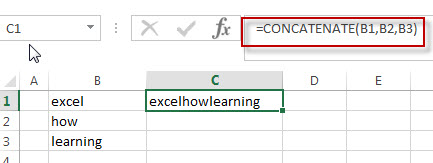Note:  If you want to add a space between the combined text strings, you can add a space character (“ “) enclosed in quotation marks. Just like the below formula:

```=CONCATENATE(B1," ",B2," ",B3)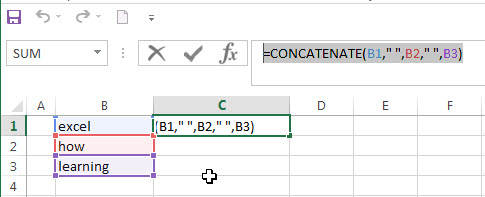```

If you want to add line break into the combined text string, you can use the CHAR function within the CONCATENATE function, just use the below formula:

`=CONCATENATE(B1,CHAR(10),B2,CHAR(10),B3)`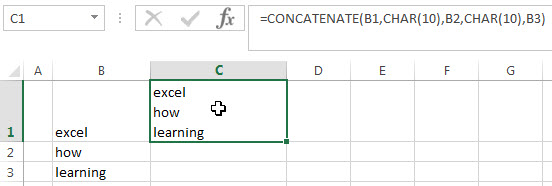## Combine text using TEXTJOIN function

If you are using the excel 2016, then you can use a new function TEXTJOIN function to combine text from multiple cells, it is only available in EXCEL 2016.  It can join the text from two or more text strings or multiple ranges into one string and also can specify a delimiter between the combined text strings. Just like the below formula:

`=TEXTJOIN(" ",TRUE,B1:B3)`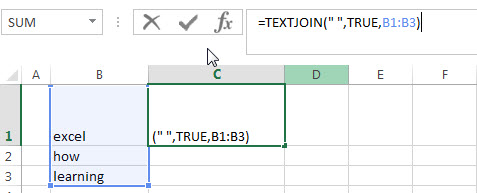### Related Formulas

• Remove Numeric Characters from a Cell
If you want to remove numeric characters from alphanumeric string, you can use the following complex array formula using a combination of the TEXTJOIN function, the MID function, the Row function, and the INDIRECT function..…
• remove non numeric characters from a cell
If you want to remove non numeric characters from a text cell in excel, you can use the array formula:{=TEXTJOIN(“”,TRUE,IFERROR(MID(B1,ROW(INDIRECT(“1:”&LEN(B1))),1)+0,””))}…

### Related Functions

• Excel Concat function
The excel CONCAT function combines 2 or more strings or ranges together.This is a new function in Excel 2016 and it replaces the CONCATENATE function.The syntax of the CONCAT function is as below:=CONCAT (text1,[text2],…)…
• Excel CHAR function
The Excel CHAR function returns the character specified by a number (ASCII Value).The CHAR function is a build-in function in Microsoft Excel and it is categorized as a Text Function. The syntax of the CHAR function is as below:=CHAR(number)….
• Excel TEXTJOIN function
The Excel TEXTJOIN function joins two or more text strings together and separated by a delimiter. you can select an entire range of cell references to be combined in excel 2016.The syntax of the TEXTJOIN function is as below:= TEXTJOIN  (delimiter, ignore_empty,text1,[text2])…

## Excel Nexted IF Functions (Statements) Tutorial (15 IF Formulas examples)

This tutorial will guide you how to use nested Excel IF function  (include multiple If statements in excel formula) with syntax and provide about 15 nested IF formula examples with the detailed explanation in Microsoft excel.

## Description

The Excel IF function perform a logical test to return one value if the condition statement is TRUE and return another value if the condition statement is FALSE. The IF function is a build-in function in Microsoft Excel and it is categorized as a Logical Function.

The excel if function just only test one condition and if you want to deal with more than one condition and return different actions depending on the result of the tests,  then you need to include several IF statements (functions) in one excel IF formula, these multiple IF statements are also called Excel Nested IF formula(Nested IFs). It’s also similar with IF-THEN-ELSE statement.

The nested IF function is formed by multiple if statements within one Excel if function. This excel nested if statement makes it possible for a single formula to take multiple actions.

The most recent versions of Excel (Excel 2016, Excel 2013, Excel 2010 and Excel 2007) allow 64 IF functions (statements) in one formula, and it was only possible up to 7 nested IF functions (statements) in Excel 2003 and lower version.

## Syntax

The syntax of Nested IF function is as below:

`=IF(Condition_1,Value_if_True_1,IF(Condition_2,Value_if_True_2,Value_if_False_2))`

Where the Nested IF function argument is:

Condition_1 –  The condition that you want to test  in the first IF statement.

Value_if_True_1 – The value that is returned if first IF statement is True. If the condition_1 return False, then move into the next IF function.

Condition_2 – The condition that you want to test in the second IF statement.

Value_if_True_2 – The value that is returned if second IF statement is True.

Value_if_False_2 – The value is returned if second IF statement is False.

This is equivalent to the following IF THEN ELSE statement:

```IF Condition_1 THEN
Value_if_True_1
ELSEIF Condition_2
Value_if_True_2
ELSE
Value_if_False_2
END IF```

## Examples of Nested IF function (Statement) in Excel

The below examples will show you how to use Excel Nested IF function with the detailed explanation of their syntax and logic.

Example 1#  The most basic Nested IF function with one level of nesting

If you want to write a nested if function to test the following calculation logic for assigning value in the cell A1.

```IF A1 =="excelhow" THEN
return "excel"
ELSEIF A1 == "excelhow.net" THEN
return "MS excel"
ELSE
return "MS"
END IF```

we can write a nested IF function based on the above logic as follows:

`=IF(A1="excelhow", "excel", IF(A1="excelhow.net","MS excel","MS"))`

In above Nested IF formula, the nested if function is shown with bold style and it is inside the outer IF function. we can see that if A1 is not equal to the “excelhow“, then the second nested  IF function will be test. and if second IF condition statement return FALSE, then the entire IF function will return “MS” value.

Example 2#  The Nested IF function with two levels of nesting

Assuming that you want to test more than one condition statement in the above nested if function, add one condition to test if the value of the cell A1 reference is equal to “www.excelhow.net” , If TRUE, then return “Microsot excel“.

The calculation Logic is as below:

```IF A1 =="excelhow" THEN
return "excel"
ELSEIF A1 == "excelhow.com" THEN
return "MS excel"
ELSEIF A1 == "www.excelhow.net" THEN
return "Microsoft excel"
ELSE
return "MS"
END IF```

we can add one more IF statement inside the second IF function in the above excel nested if formula in example1. let’s see the below nested if function with tow level nesting:

`=IF(A1="excelhow", "excel", IF(A1="excelhow.com","MS excel",IF(A1="www.excelhow.net","Microsoft excel","MS")))`

In the above nested excel IF formula, the first nested if function is marked with red color, and the second nested excel if function is marked with blue color.

If the both first and second conditions are False and the third IF condition will be check, IF A1 is equal to “www.excelhow.net” , then return “Microsoft excel“, or the entire nested IF formula will return “MS“.

Example 3#  Describes the each IF function contained in the nested IF function

​We will use one typical example of excel nested if function to describe each IF function included in the nested if function.

Assuming that you need to assign a grade based on a score with the following test conditions:

Let’s write a nested if function based on the above logic as follows:

`=IF(A1>=80, "excellent", IF(A1>=60, "good", IF(A1>0, "bad", "no valid score")))`

For the above excel if formula, lets describe it for each IF function statement.

1# IF Cell A1 is greater than or equal to 80, then the formula will return “excellent” or move to the second If function.

2# If Cell A1 is greater than or equal to 60, then the formula will return  “good” or move to the third IF function

3# IF Cell A1 is greater than 0, then the formula will return “bad”, or the IF function will return “no valid score”.

Example 4#  Describes each If function in the excel Nested IF Statement (another simple example of if function)

Let’s describe the below Nested IF Function example:

`=IF(A1<=6,60, IF(A1<=8,80,IF(A1<=10,100,200)))`

a) If cell A1 is equal to 6 or less than 6, then return value 60 in cell C1. Let’s see below screenshot.

b) If Cell A1 is greater than 6 and less or equal to 8, then retrun value 80 in Cell C1.

c) If cell A1 is greater than 8 and less than or equal to 10, then return value 100 in cell C1.

d) If cell A1 is greater than 10 , then the Nested if function will return the last value “200”in cell C1.

Example 5#  Excel Nested IF function with arithmetic operator (+, -, * , /)

Assuming that you want to write a Nested If function to reflect the following logic tasks:

a) IF Cell A1 is less than 10, then multiply by 10.

b) IF Cell A1 is greater than or equal to 10 but less than 20, then add 20

c) IF Cell A1 is greater than or equal to 20 but less than 30, then minus 20

d) IF Cell A1 is greater than or equal to 30 but less than 50, then divided by 20

The nested IF formula is as follows:

`=IF(A1<10,A1*10,IF(A1<20,A1+20,IF(A1<30,A1-20,IF(A1<50,A1/20))))`

a1) if Cell A1 is less 10 (A1=5), then the first If condition matched and will take multiply action, A1 * 10=5*10=50, so it will return 50 in the cell C1

b1) if Cell 10<=A1<20 (A1=15), then the second if condition matched and will take add action, A1+20=15+20=35, so it will return 35 in the cell C1.

c1) if Cell 20<=A1<30(A1=25), then the third if condition matched and will take minus action, A1-20=25-20=5, so it will return 5 in the cell C1.

d1) if Cell 30<=A1<50 (A1=35), then the forth if condition matched and will take divide action, A1/20=35/20=1.75, so it will return 1.75 in the cell C1.

Example 6#  Excel Nested IF function with logical function –AND

Assuming that you need a nested if function to reflect the following logic:

a) IF A1+B1 is less than 10, then return 10

b) IF A1+B1 is greater than 10 but less than or equal to 20, then return 20

c) IF A1+B1 is greater than 20 but less than or equal to 30, then return 30.

d) IF A1+B1 is greater than 30, then return 200.

Let’s write the following nested IF formula in the cell C1:

`=IF(A1+B1<10,10, IF(A1+B1<=20,20, IF(A1+B1<=30,30,200)))`

The above formula just use basic nested IF function syntax, we also can use logic function to re-write it, the nested if formula with AND function is as follows:

`=IF((A1+B1)<10,10,IF(AND((A1+B1)>10,(A1+B1)<=20),20, IF(AND((A1+B1)>20,(A1+B1)<=30),30,200)))`

The above nested IF formula combined with two AND function.

In the second IF Statement, AND((A1+B1)>10,(A1+B1)<=20) will check if 10<A1+B1<=20, If TRUE, then the formula will return 20.

In the third IF Statement, AND((A1+B1)>20,(A1+B1)<=30) will check if 20<A1+B1<=30, If TRUE, then the formula will return 30.

Example 7# Excel Nested IF function with logical function –OR

Assuming that you need a nested if function to reflect the following logic:

a) IF Cell A1=5, return A1/B1

b) IF Cell A1=10, return A2/B2

c) IF Cell A1=15 or A1=20, return A3/B3

In Cell C1, we can write the below nested if formula based on the above conditions.

`=IF(A1=5,A1/B1,IF(A1=10,A2/B2,IF(OR(A1=15,A1=20),A3/B3)))`

One OR function be used in the above excel nested if function, it will check if A1=15 or A1=20, if TRUE, then return A3/B3.

Example 8#  Excel nested if function with text and logical function AND

Wrote a nested if function with text to reflect the following logic:

a) If Cell A1=”E” and Cell B1=5, then return “Excel”

b) If Cell A1=”P” and Cell B1=3, then return “PPT”

c) If Cell A1=”W” and Cell B1=4, then return “Word”

d) Else return “Access”

In Cell C1, try to enter into the following excel nested If formula with AND function:

`=IF(AND(A1="E",B1=5),"Excel", IF(AND(A1="P",B1=3),"PPT", IF(AND(A1="W",B1=4),"Word","Access")))`

Example 9#  Excel nested if function with ISBLANK function and logical function AND

a) If you want to wrote a nested if function with ISBLANK function and logical function AND to reflect the following logic:

b) If both Cell A1 and Cell B1 are empty, then return “”

c) If only Cell A1 is empty, then return B1-today()

d) If both two cells A1 and B1 are not empty, then return “excel” string.

In Cell C1, use the following excel nested If formula with ISBLANK and AND function:

`=IF(AND(ISBLANK(A1),ISBLANK(B1)),"",IF(ISBLANK(A1),B1-TODAY(),"excel"))`

Example 10#  Using nested IF functions to check grade level based on student’s score(multiple IF statements)

The logic is as below:

We will write a nested If function that reflect the above logic, and will check if the score is below 50, If TRUE, it is considered as “Fail”. If FALSE, move into the next IF statement to test if the score is between 51 and 60 and it is considered as “Grade C”. If False, we will move into another IF statement to check if the score is between 61 and 80, IF True and it is considered as “Grade B”. If FASLSE, just check the rest conditions.

We can use a nested if formula as follows:

`=IF(B2<=50,"Fail",IF(AND(B2>=51, B2<=60), "Grade C", IF(AND(B2>=61, B2<=80),"Grade B",IF(AND(B2>=81,B2<=95),"Grade A", "Grade A+"))))`

Example 11#  Nested IF function for checking two Empty Cells

Let’s see the below image a product table of a company (need to create an excel table firstly):

a) If we need to check both “Price” cell and “Quantity” cell are empty, If True, then return empty. If the only “price” cell is empty only, IF True, return empty.

b) If the only “Quantity” cell is empty, IF True, return empty.

c) If both “price” and “Quantity” are not empty, then return multiply Price * Quantity as subtotal value.

So To check both “Price” and Quantity cells, we can use table header name as condition variable to test each Price cells or Quantity cells, so we can write the nested if formula as follows:

`=IF([Price]="","",IF([Quantity]="","",[Price]*[Quantity]))`

Just using the above excel if formula in the subtotal cells, the formula will check the first IF statement if Price Cell is empty, IF TRUE, then will return empty (“”) in the subtotal cell. IF FALSE, then move to the next IF statement and so on. Last, IF neither cell is empty, then will return the value of multiply [Price]*[Quantity] in subtotal cell.

Of course, we can use another nested if function to achieve the above calculation logic (easy to understand).

`=IF(ISBLANK(B2),"",IF(ISBLANK(C2),"", B2*C2))`

OR

`=IF(B2="","",IF(C2="","", B2*C2))`

## Nested IF Functions Order

There is one important thing that need us keep in mind when write Excel Nested IF Function, it is the order of nested IF function. It can nested up to 64 If statements, and how to order multiple IF condition statements, it is key point. Or the wrong result will be returned. The point is that excel nested if function will test the first if condition in the order, once any condition is met, and the subsequent if conditions will not be checked.

So let’s remember the below rules while writing excel nested if function:

• The most important condition First or Harder Test First

Let’s see the below example what it means:

Example 12#

There are two test conditions in the following excel nested if function:

`=IF(B1>=90,"excellent",IF(B1>=60,"good","bad"))`

When using this formula in the cell B3, If the amount in cell B1 is 95, then “excellent” would be returned. because it is greater than 90. And the second IF condition will not be evaluated.

However, if the order of nested if statment are reversed as follows:

`=IF(B1>=60,"good",IF(B1>=90,"excellent","bad"))`

The above formula would check for the condition B1>=60 first, if the amount in cell B1 is 95,  then the value “good” would be returned in cell B3. Because the Cell B1 match the first test condition, and it will not check the second if condition and will return the incorrect result.

## Nested IF Function Alternatives

To make your excel formulas more efficiency and fast, you can try to use the following alternatives to excel nested if function.

1)
Excel nested if function can be easily replaced with the VLOOUP, Lookup, INDEX/MATCH or CHOOSE functions.

Example 13#  Use VLookup function instead of nested IF function

Nested IF function:

`=IF(D1=39,"excelhow.net", IF(D1=45,"two",IF(D1=34,"google.com","")))`

Vlookup function:

`=VLOOKUP(39,A1:B3,2,FALSE)`

Example 14#  Use CHOOSE function instead of nested if function

Nested If unction:

`=IF(B1>=60,"good","bad")`

Using CHOOSE function as follows:

`=CHOOSE(IF(B1<60,1,2),"bad","good")`

2) Use IFS instead of nested if function

3) Use the CONCATENATE function or the concatenate operator (&).

Example 15#

Nested IF function:

`=IF(B1="A","excellent",IF(B1="B","good","bad"))`

Using CONCATENATE function as follows:

`=CONCATENATE(IF(B1=”A”,”excellent”,””),IF(B1=”B”,”good”,””),IF(B1=”C”,”bad”))`

Question1: Is there any tool to help write Excel formulas and nested Ifs?

This is an Excel formula with nested IF statements:

`=IF((B2="East"),4,IF((B2="West"),3,IF((B2="North"),2,IF((B2="South"),1,""))))`

To essentially accomplish this:

```If cell B2 = "East"
return "4"
ElseIf cell B2 = "West"
return "3"
ElseIf cell B2 = "North"
return "2"
ElseIf cell B2 = "South"
return "1"
Else
return ""```

Can Excel formulas be written in such a “more readable” manner and converted to the official syntax? Is there any tool to help write Excel formulas?

Answer: Excel Formula Formatter add-in by Rob van Gelder, mentioned at Daily Dose of Excel.

Excel’s formula bar ignores line feeds and white space, so you can Alt+Enter and spacebar to format the formulas however you like. I’ve tried it and I quickly stopped doing it. Too much spacebar-ing, especially if you need to edit.

Question2: I am working on an excel file, and i am trying to use a nested if formula to achieve what i would like.

i have two columns:A B.  And the condition is this: if the value in a2=a3, then check if the minus of b2 and b3 is certain value, and if it is, put a yes, else put a no. this will iterate till the end of the excel file.

so far here is what i have. not sure how to use the excel formulas. any help is much appreciated.

`if(a2=a3,b2-b3=5 or b2-b3=-5 or b2-b3=20 or b2-b3=-20, "yes", "no")`

Answer: you should be able to use the OR function within your nested if formula to test for “B2-B3=5,B2-B3=-5,B2-B3=20,B2-B3=-20” as follows:

`=IF(A2=A3,IF(OR(B2-B3=5,B2-B3=-5,B2-B3=20,B2-B3=-20),"yes","no"),"no")`

### Related Functions

• Excel IF function
The Excel IF function perform a logical test to return one value if the condition is TRUE and return another value if the condition is FALSE….
• Excel And function
The Excel AND function returns TRUE if all of arguments are TRUE, and it returns FALSE if any of arguments are FALSE…
• Excel Concat Function
The excel CONCAT function combines 2 or more strings or ranges together. The syntax of the CONCAT function is as below: =CONCAT (text1,[text2],…) …
• Excel Vlookup Function
The Excel VLOOKUP function lookup a value in the first column of the table and return the value in the same row based on index_num position.The syntax of the VLOOKUP function is as below:= VLOOKUP (lookup_value, table_array, column_index_num,[range_lookup])…
• Excel Choose Function
The Excel CHOOSE function returns a value from a list of values. The CHOOSE function is a build-in function in Microsoft Excel and it is categorized as a Lookup and Reference Function.The syntax of the CHOOSE function is as below:=CHOOSE (index_num, value1,[value2],…)…
• Excel ISBLANK function
The Excel ISBLANK function returns TRUE if the value is blank or null.The syntax of the ISBLANK function is as below:= ISBLANK (value)…

## Excel Concat Function

This post will guide you how to use Excel CONCAT function with syntax and examples in Microsoft excel.

### Description

The excel CONCAT function combines 2 or more strings or ranges together.

This is a new function in Excel 2016 and it replaces the CONCATENATE function. However, the CONCATENATE function is still available for earlier version of Microsoft Excel.
​Note: The CONCAT function do not support the delimiter or Ignore Empty arguments.

The CONCAT function is a build-in function in Microsoft Excel and it is categorized as a Statistical Function.

The CONCAT function is available in Excel 2016. If you are using Excel 2013 or older version, just use the CONCATENTATE function instead.

### Syntax

The syntax of the CONCAT function is as below:

=CONCAT (text1,[text2],…)

Where the CONCAT  function arguments are:
text1 – the strings that you want to combine together.
text2 – the additional strings to be joined

### Example

#1 To join the strings from B1 to B5, just using the following excel formula: =CONCAT(B1,B2,B3,B4,B5)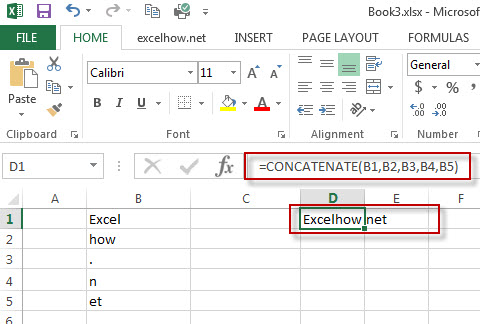#2 To join three strings: the string in Cell B1, a string with a space character and the string value in cell B2